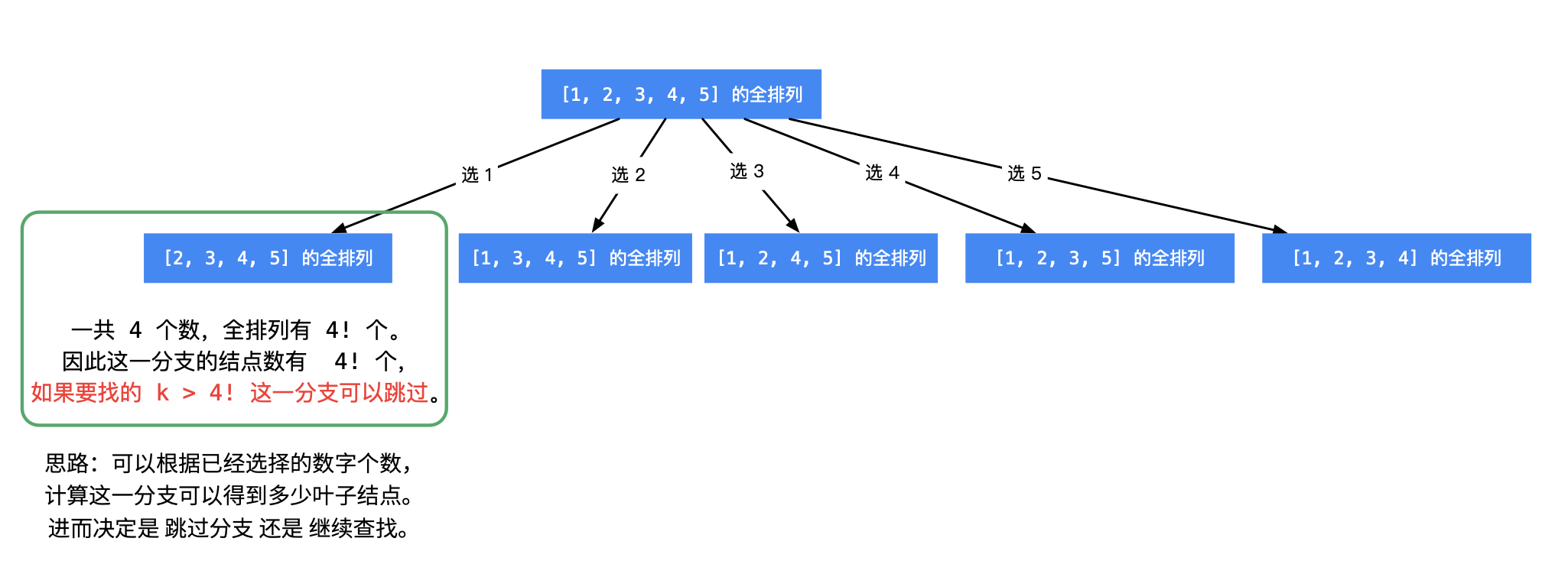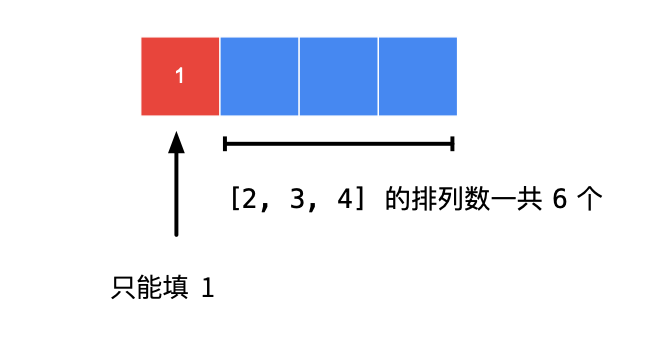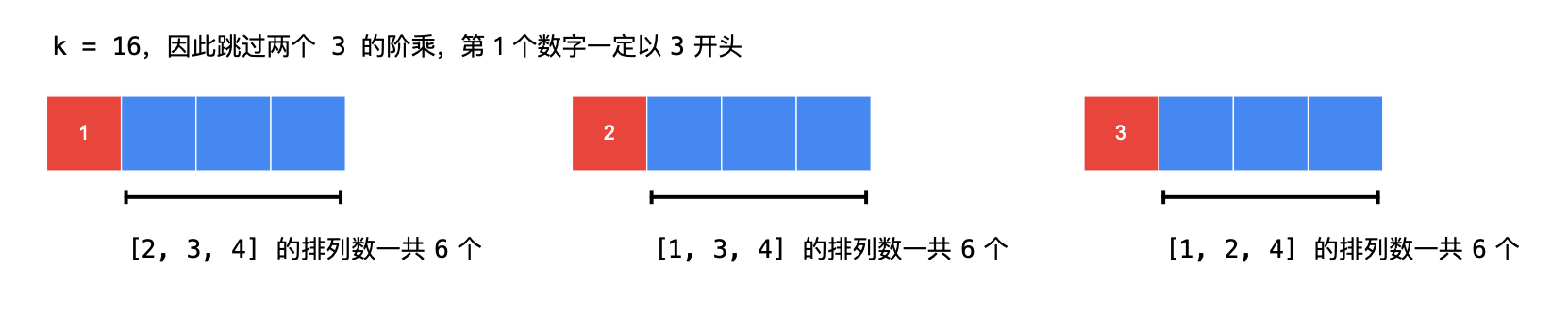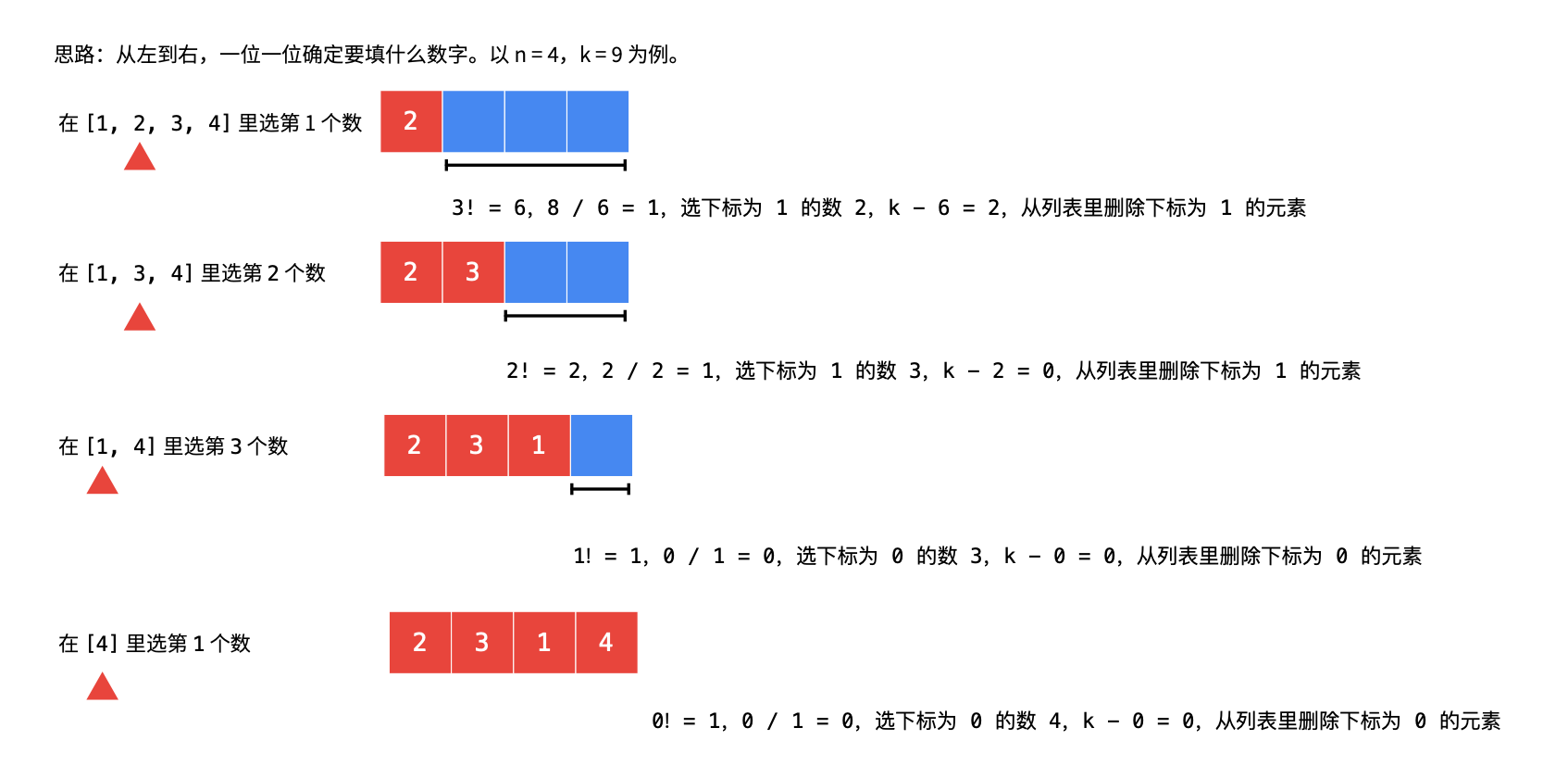# 31. 下一个排列

## 题目

### 例子

输入：nums = [1,2,3]



输入：nums = [3,2,1]



输入：nums = [1,1,5]



1 <= nums.length <= 100

0 <= nums[i] <= 100

## 思路

1. 如何判断有木有下一个呢？只要存在a[i-1] < a[i]的升序结构，就有，而且我们应该从右往左找

2. 当发现a[i-1] < a[i]的结构时，从在[i, ∞]中找到最接近a[i-1]并且又大于a[i-1]的数字，由于降序，从右往左遍历即可得到k

## 算法

1） 首先从后向前查找第一个顺序对 (i,i+1) ，满足 a[i] < a[i+1]。这样「较小数」即为 a[i]。此时 [i+1,n) 必然是下降序列。

2) 如果找到了顺序对，那么在区间 [i+1,n) 中从后向前查找第一个元素 jj 满足 a[i] < a[j]。这样「较大数」即为 a[j]a[j]。

3) 交换 a[i] 与 a[j]，此时可以证明区间 [i+1,n) 必为降序。我们可以直接使用双指针反转区间 [i+1,n) 使其变为升序，而无需对该区间进行排序。

> 如果在步骤 1 找不到顺序对，说明当前序列已经是一个降序序列，即最大的序列，我们直接跳过步骤 2 执行步骤 3，即可得到最小的升序序列。

## java 实现

java
class Solution {
public void nextPermutation(int[] nums) {
int i = nums.length - 2;
while (i >= 0 && nums[i] >= nums[i + 1]) {
i--;
}
if (i >= 0) {
int j = nums.length - 1;
while (j >= 0 && nums[i] >= nums[j]) {
j--;
}
swap(nums, i, j);
}
reverse(nums, i + 1);
}

public void swap(int[] nums, int i, int j) {
int temp = nums[i];
nums[i] = nums[j];
nums[j] = temp;
}

public void reverse(int[] nums, int start) {
int left = start, right = nums.length - 1;
while (left < right) {
swap(nums, left, right);
left++;
right--;
}
}
}


# 46. 全排列 permutations

## 题目

### 例子

输入：nums = [1,2,3]



输入：nums = [0,1]



输入：nums = 



### 提示：

1 <= nums.length <= 6

-10 <= nums[i] <= 10

nums 中的所有整数 互不相同

## 思路

> [回溯算法-backtrack](https://houbb.github.io/2020/01/23/data-struct-learn-07-base-backtracking)

## java 实现

java
public List<List<Integer>> permute(int[] nums) {
// 这个个数实际上可以预测：就是 nums ! 阶乘。
// 实测优化效果不明显
final int len = nums.length;
List<List<Integer>> results = new ArrayList<>();
// 避免扩容
List<Integer> tempList = new ArrayList<>(len);
backtrack(results, tempList, nums);
return results;
}

private void backtrack(List<List<Integer>> results, List<Integer> tempList, int[] nums) {
// 什么时候停止
final int len = nums.length;

// 满足条件
if(tempList.size() == len) {
} else {
// 回溯
for (int current : nums) {
// 元素不能重复
if (tempList.contains(current)) {
continue;
}
// 下一个元素
backtrack(results, tempList, nums);
// 回溯
tempList.remove(tempList.size() - 1);
}
}
}


# 47. 全排列 II permutations-ii

## 题目

### 例子

输入：nums = [1,1,2]

[[1,1,2],
[1,2,1],
[2,1,1]]


输入：nums = [1,2,3]



1 <= nums.length <= 8

-10 <= nums[i] <= 10

## V1-复用 46

### 思路

### java 实现

java
class Solution {

public List<List<Integer>> permuteUnique(int[] nums) {
// 这个个数实际上可以预测：就是 nums ! 阶乘。
// 实测优化效果不明显
final int len = nums.length;
boolean[] used = new boolean[len];
List<List<Integer>> results = new ArrayList<>();

// 避免扩容
List<Integer> tempList = new ArrayList<>(len);
backtrack(results, tempList, nums, used);

// 过滤重复的值
return filter(results);
}

private void backtrack(List<List<Integer>> results, List<Integer> tempList, int[] nums,
boolean[] used) {
// 什么时候停止
final int len = nums.length;
if(tempList.size() == len) {
} else {
// 回溯
for (int i = 0; i < nums.length; i++) {
// 元素不能重复使用
if (used[i]) {
continue;
}

used[i] = true;

// 下一个元素
backtrack(results, tempList, nums, used);

// 回溯
tempList.remove(tempList.size() - 1);
used[i] = false;
}
}
}

private List<List<Integer>> filter(List<List<Integer>> all) {
List<List<Integer>> results = new ArrayList<>();

for(List<Integer> list : all) {
if(!results.contains(list)) {
}
}

return results;
}

}


### 效果

Runtime: 857 ms, faster than 5.26% of Java online submissions for Substring with Concatenation of All Words.
Memory Usage: 47.4 MB, less than 22.52% of Java online submissions for Substring with Concatenation of All Words.


## V2-剪枝

### 思路

// 如果有重复的元素，就会导致重复。（需要数组进行排序）
// 1_a 1_b 2   第一次 1_a 1_b 2
// 如果出现 1_b 1_a 2 实际上就重复了。（需要跳过的 case）
if(i > 0 && nums[i] == nums[i-1] && !used[i-1]) {
continue;
}


### java 实现

class Solution {
/**
* 解题思路：回溯算法
*
* 【优化思路】
* 避免数组扩容
*
* @param nums 数字
* @return 结果
* @since v46
*/
public List<List<Integer>> permuteUnique(int[] nums) {
Arrays.sort(nums);

// 这个个数实际上可以预测：就是 nums ! 阶乘。
// 实测优化效果不明显
final int len = nums.length;
List<List<Integer>> results = new ArrayList<>();

boolean[] used = new boolean[len];
// 避免扩容
List<Integer> tempList = new ArrayList<>(len);
backtrack(results, tempList, nums, used);
return results;
}

private void backtrack(List<List<Integer>> results, List<Integer> tempList, int[] nums,
boolean[] used) {
// 什么时候停止
final int len = nums.length;
if(tempList.size() == len) {
} else {
// 回溯
for (int i = 0; i < len; i++) {
// 已经被使用的，不能重复使用。
// 如果没有重复的元素，这样就要够了
if(used[i]) {
continue;
}

// 如果有重复的元素，就会导致重复。（需要数组进行排序）
// 1_a 1_b 2   第一次 1_a 1_b 2
// 如果出现 1_b 1_a 2 实际上就重复了。（需要跳过的 case）
if(i > 0 && nums[i] == nums[i-1] && !used[i-1]) {
continue;
}

int current = nums[i];
used[i] = true;

// 下一个元素
backtrack(results, tempList, nums, used);

// 回溯
used[i] = false;
tempList.remove(tempList.size() - 1);
}
}
}
}


### 效果

Runtime: 1 ms, faster than 100% of Java online submissions for Substring with Concatenation of All Words.
Memory Usage: 39.4 MB, less than 100% of Java online submissions for Substring with Concatenation of All Words.


# 60. 排列序列 permutation sequence

## 题目

"123"
"132"
"213"
"231"
"312"
"321"


### 例子

输入：n = 3, k = 3



输入：n = 4, k = 9



输入：n = 3, k = 1



1 <= n <= 9

1 <= k <= n!

## v1-基本方式

### java 实现

    public String getPermutation(int n, int k) {
List<List<Integer>> all = new ArrayList<>();

List<Integer> tempList = new ArrayList<>();
backtrack(all, tempList, n, k, 1);

// 返回列表中的最后一个。
List<Integer> integers = all.get(k-1);
StringBuilder stringBuilder = new StringBuilder();
for(Integer i : integers) {
stringBuilder.append(i);
}
return stringBuilder.toString();
}

// 如何可以簡化這個操作呢？
// 其實我們只關心第 K 個元素
private void backtrack(List<List<Integer>> all, List<Integer> tempList,
int n, int k, int start) {
if(tempList.size() == n) {
// 满了

// 如果大小已经够了，直接剪枝
if(all.size() >= k) {
return;
}
}

for(int i = 1; i <= n; i++) {
// 跳过重复的元素
if(tempList.contains(i)) {
continue;
}

// 下一个元素
backtrack(all, tempList, n, k, start+1);
// 移除，回溯
tempList.remove(tempList.size()-1);
}
}


## V2-回溯剪枝

### 思路### 阶乘

/**
* 计算阶乘数组
*
* @param n
*/
private void calculateFactorial(int n) {
factorial = new int[n + 1];
factorial = 1;
for (int i = 1; i <= n; i++) {
factorial[i] = factorial[i - 1] * i;
}
}


### java 实现

import java.util.Arrays;

public class Solution {

/**
* 记录数字是否使用过
*/
private boolean[] used;

/**
* 阶乘数组
*/
private int[] factorial;

private int n;
private int k;

public String getPermutation(int n, int k) {
this.n = n;
this.k = k;
calculateFactorial(n);

// 查找全排列需要的布尔数组
used = new boolean[n + 1];
Arrays.fill(used, false);

StringBuilder path = new StringBuilder();
dfs(0, path);
return path.toString();
}

/**
* @param index 在这一步之前已经选择了几个数字，其值恰好等于这一步需要确定的下标位置
* @param path
*/
private void dfs(int index, StringBuilder path) {
if (index == n) {
return;
}

// 计算还未确定的数字的全排列的个数，第 1 次进入的时候是 n - 1
// 直接根据计算好的排列，计算当前的 count
int cnt = factorial[n - 1 - index];
for (int i = 1; i <= n; i++) {
if (used[i]) {
continue;
}
if (cnt < k) {
k -= cnt;
continue;
}
path.append(i);
used[i] = true;
dfs(index + 1, path);
// 注意 1：不可以回溯（重置变量），算法设计是「一下子来到叶子结点」，没有回头的过程
// 注意 2：这里要加 return，后面的数没有必要遍历去尝试了
return;
}
}

/**
* 计算阶乘数组
*
* @param n
*/
private void calculateFactorial(int n) {
factorial = new int[n + 1];
factorial = 1;
for (int i = 1; i <= n; i++) {
factorial[i] = factorial[i - 1] * i;
}
}
}


## V3-有序数组（链表）模拟

### 思路### 算法

1) 把候选数放在一个 有序列表 里，从左到右根据「剩下的数的阶乘数」确定每一位填谁，公式 k / (后面几位的阶乘数) 的值 恰好等于候选数组的下标； 选出一个数以后，k 就需要减去相应跳过的阶乘数的倍数；

2) 已经填好的数需要从候选列表里删除，注意保持列表的有序性（因为排列的定义是按照字典序）；

3) 由于这里考虑的是下标，第 k 个数，下标为 k - 1，一开始的时候，k–。

4) 每次选出一个数，就将这个数从列表里面拿出。这个列表需要支持频繁的删除操作，因此使用双链表。在 Java 中 LinkedList 就是使用双链表实现的。### java 实现

import java.util.LinkedList;
import java.util.List;

public class Solution {

public String getPermutation(int n, int k) {
// 注意：相当于在 n 个数字的全排列中找到下标为 k - 1 的那个数，因此 k 先减 1
k --;

int[] factorial = new int[n];
factorial = 1;
// 先算出所有的阶乘值
for (int i = 1; i < n; i++) {
factorial[i] = factorial[i - 1] * i;
}

// 这里使用数组或者链表都行
for (int i = 1; i <= n; i++) {
}

StringBuilder stringBuilder = new StringBuilder();

// i 表示剩余的数字个数，初始化为 n - 1
for (int i = n - 1; i >= 0; i--) {
int index = k / factorial[i] ;
stringBuilder.append(nums.remove(index));
k -= index * factorial[i];
}
return stringBuilder.toString();
}
}


# 开源地址

https://github.com/houbb/leetcode

## 参考资料

https://leetcode.cn/problems/next-permutation/solution/xia-yi-ge-pai-lie-by-leetcode-solution/

https://leetcode.cn/problems/permutations/

https://leetcode.cn/problems/permutations-ii/

https://leetcode.cn/problems/permutation-sequence/solution/di-kge-pai-lie-by-leetcode-solution/

https://leetcode.cn/problems/permutation-sequence/solution/hui-su-jian-zhi-python-dai-ma-java-dai-ma-by-liwei/